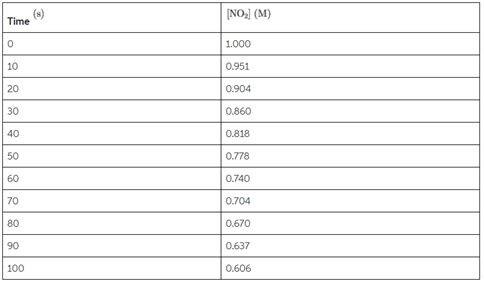Problem: Consider the following reaction: NO2(g) → NO(g) + 1/2 O2(g). The following data were collected for the concentration of NO2 as a function of time. Express your answer using two significant figures.a. What is the average rate of the reaction between 10 and 20?b. What is the average rate of the reaction between 50 and 60 ?c. What is the rate of formation of O2 between 50 and 60 ?

FREE Expert Solution
80% (237 ratings)
Problem Details

Consider the following reaction: NO2(g) → NO(g) + 1/2 O2(g). The following data were collected for the concentration of NO2 as a function of time. Express your answer using two significant figures.

a. What is the average rate of the reaction between 10 and 20?

b. What is the average rate of the reaction between 50 and 60 ?

c. What is the rate of formation of Obetween 50 and 60 ?What scientific concept do you need to know in order to solve this problem?

Our tutors have indicated that to solve this problem you will need to apply the Average Rate of Reaction concept. You can view video lessons to learn Average Rate of Reaction Or if you need more Average Rate of Reaction practice, you can also practice Average Rate of Reaction practice problems .

What is the difficulty of this problem?

Our tutors rated the difficulty of Consider the following reaction: NO2(g) → NO(g) + 1/2 O2(g).... as high difficulty.

How long does this problem take to solve?

Our expert Chemistry tutor, Dasha took 5 minutes to solve this problem. You can follow their steps in the video explanation above.

What professor is this problem relevant for?

Based on our data, we think this problem is relevant for Professor Deshmukh's class at UCSD.